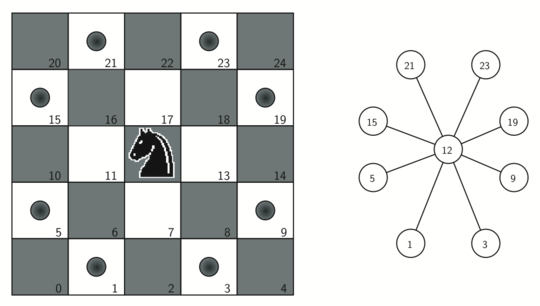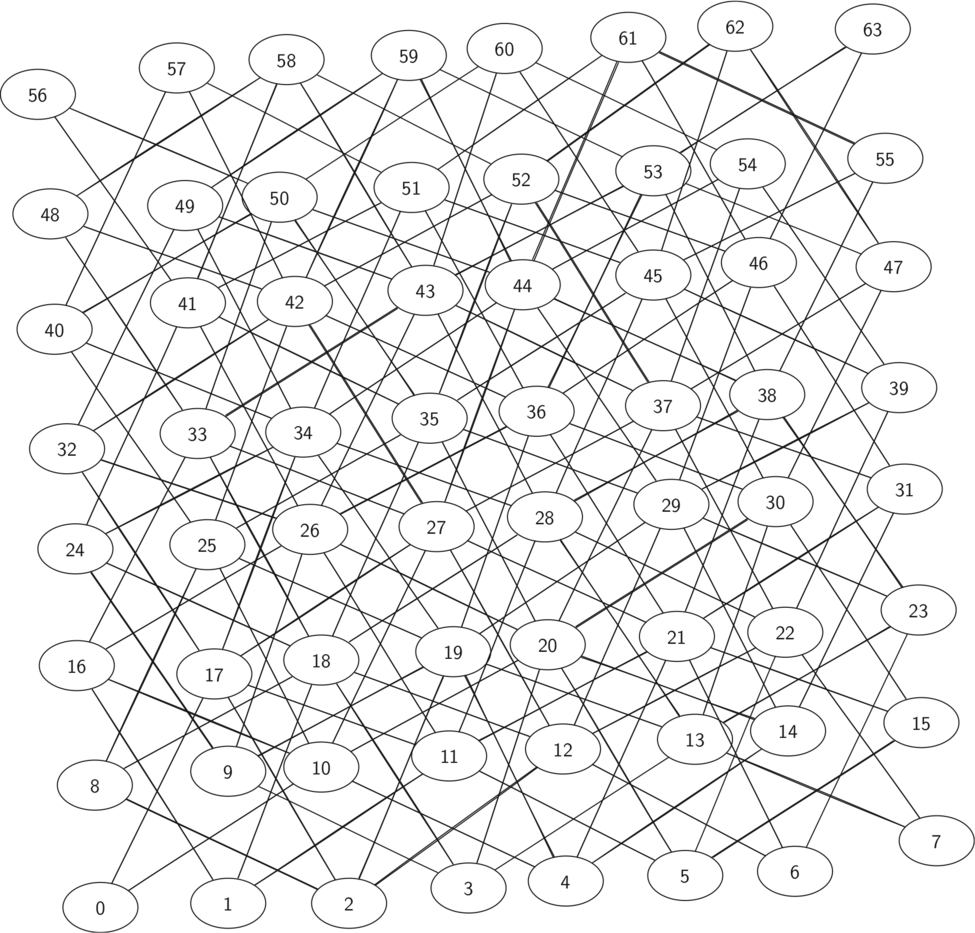# 8.12. Building the Knight’s Tour Graph¶

To represent the knight’s tour problem as a graph we will use the following two ideas: Each square on the chessboard can be represented as a node in the graph. Each legal move by the knight can be represented as an edge in the graph. Figure 1 illustrates the legal moves by a knight and the corresponding edges in a graph.Figure 1: Legal Moves for a Knight on Square 12, and the Corresponding Graph

To build the full graph for an n-by-n board we can use the Python function shown in Listing 1. The knightGraph function makes one pass over the entire board. At each square on the board the knightGraph function calls a helper, genLegalMoves, to create a list of legal moves for that position on the board. All legal moves are then converted into edges in the graph. Another helper function posToNodeId converts a location on the board in terms of a row and a column into a linear vertex number similar to the vertex numbers shown in Figure 1.

Listing 1

from pythonds.graphs import Graph

def knightGraph(bdSize):
ktGraph = Graph()
for row in range(bdSize):
for col in range(bdSize):
nodeId = posToNodeId(row,col,bdSize)
newPositions = genLegalMoves(row,col,bdSize)
for e in newPositions:
nid = posToNodeId(e,e,bdSize)
return ktGraph

def posToNodeId(row, column, board_size):
return (row * board_size) + column


The genLegalMoves function (Listing 2) takes the position of the knight on the board and generates each of the eight possible moves. The legalCoord helper function (Listing 2) makes sure that a particular move that is generated is still on the board.

Listing 2

def genLegalMoves(x,y,bdSize):
newMoves = []
moveOffsets = [(-1,-2),(-1,2),(-2,-1),(-2,1),
( 1,-2),( 1,2),( 2,-1),( 2,1)]
for i in moveOffsets:
newX = x + i
newY = y + i
if legalCoord(newX,bdSize) and \
legalCoord(newY,bdSize):
newMoves.append((newX,newY))
return newMoves

def legalCoord(x,bdSize):
if x >= 0 and x < bdSize:
return True
else:
return False


Figure 2 shows the complete graph of possible moves on an eight-by-eight board. There are exactly 336 edges in the graph. Notice that the vertices corresponding to the edges of the board have fewer connections (legal moves) than the vertices in the middle of the board. Once again we can see how sparse the graph is. If the graph was fully connected there would be 4,096 edges. Since there are only 336 edges, the adjacency matrix would be only 8.2 percent full.Figure 2: All Legal Moves for a Knight on an $$8 \times 8$$ Chessboard